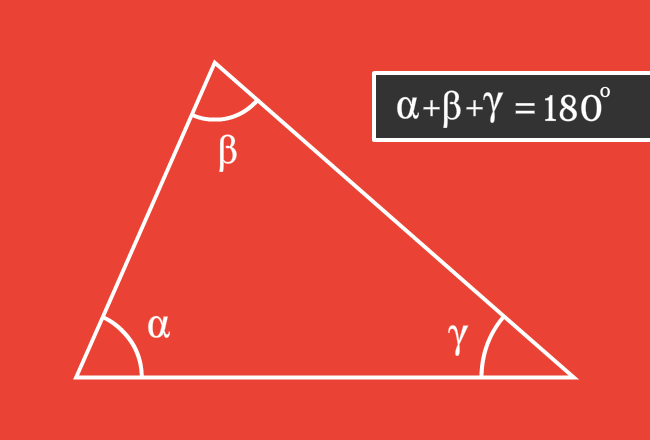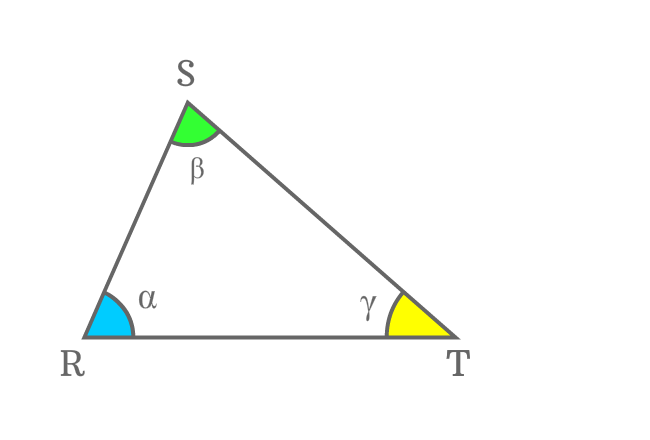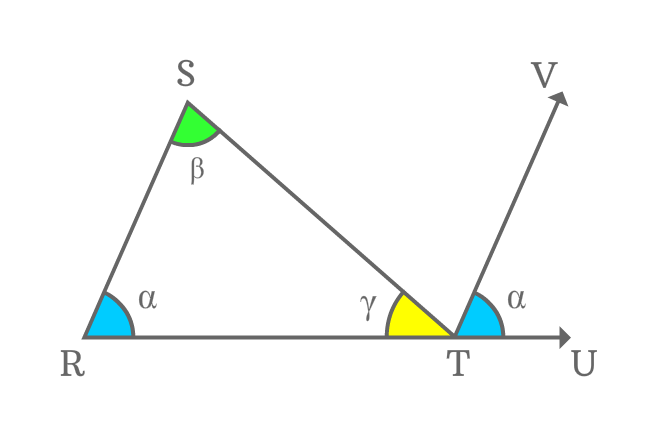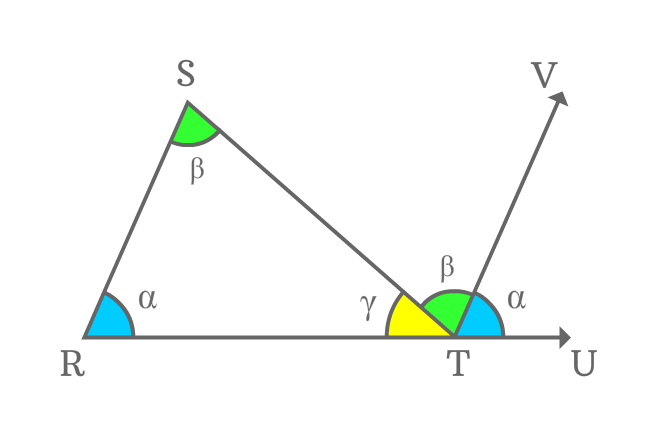# Sum of angles of a triangle

## Property

The sum of all three interior angles in a triangle is $180^\circ$.Three interior angles are formed internally by the intersection of every two sides of a triangle. The addition of all three angles is always equal to $180^\circ$ geometrically.

If, $\alpha$, $\beta$ and $\gamma$ are three interior angles in a triangle, then

$\alpha+\beta+\gamma = 180^\circ$

This basic geometrical property of a triangle is often used as a formula in geometry in some special cases

### Proof

There are three geometrical steps involved for proving that the sum of interior angles in a triangle is equal to $180^\circ$.

$\Delta RST$ is a triangle and its angles are $\alpha$, $\beta$ and $\gamma$.

#### Draw a Corresponding Angle to a side1. Extend the side $\small \overline{RT}$ from point $\small T$ and it passes through the point $\small U$.
2. Now, draw a parallel line $\small \overline{TV}$ to the side $\small \overline{RS}$ at point $\small T$. Thus, $\small \angle VTU$ is formed externally.
3. $\small \angle SRT$ and $\small \angle VTU$ are corresponding angles, which are actually formed by the intersection of parallel lines ($\small \overline{SR}$ and $\small \overline{TV}$) and their transversal line $\small \overline{RU}$. Geometrically, the corresponding angles are equal. Therefore, $\small \angle VTU = \angle SRT = \alpha$.

#### Identify Alternate Interior AnglesThe side $\small \overline{ST}$ is another transversal of the parallel lines $\small \overline{SR}$ and $\small \overline{TV}$. In this case, $\small \angle RST$ and $\small \angle STV$ are interior alternate angles.

It is proved that when two parallel lines are intersected by their transversal, the interior alternate angles are equal.

$\small \angle RST = \angle STV = \beta$

Therefore, $\small \angle STV$ is also equal to $\beta$ geometrically.

#### Use concept of Straight Angle

$\small \angle RTS$, $\small \angle STV$ and $\small \angle VTU$ are three angles and sum of three interior angles is equal to $\small \angle RTU$.$\small \angle RTS + \angle STV + \angle VTU$ $\,=\,$ $\small \angle RTU$

Actually, $\small \angle RTU$ is a straight angle of the straight line $\small \overline{RU}$. Geometrically, the angle of a straight line is equal to $180^\circ$.

$\implies$ $\small \angle RTS + \angle STV + \angle VTU$ $\,=\,$ $\small \angle RTU$ $\,=\,$ $180^\circ$

$\implies$ $\gamma+\beta+\alpha$ $\,=\,$ $180^\circ$

$\,\,\, \therefore \,\,\,\,\,\, \alpha+\beta+\gamma \,=\, 180^\circ$

$\alpha$, $\beta$ and $\gamma$ are three interior angles of triangle $RST$ and it is proved that the sum of angles in a triangle is equal to $180^\circ$ geometrically.

Latest Math Topics
Jun 26, 2023
Jun 23, 2023

###### Math Questions

The math problems with solutions to learn how to solve a problem.

Learn solutions

Practice now

###### Math Videos

The math videos tutorials with visual graphics to learn every concept.

Watch now

###### Subscribe us

Get the latest math updates from the Math Doubts by subscribing us.